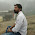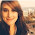# C++ Program To Swap Two Numbers Using Functions

Problem :- Write A C++ Program To Swap Two Numbers Call By Reference/Call By Value Using Functions .

Logic :- There are two method you can use to swap Two Number using function
1. Call by Reference
2. Call by Value

Call by Reference :- In Call by reference we pass address of variable in function not value of variable .

Call by Value :- In Call by Value we pass value of variable in function .

Solution :-

Method 1 :- Call by Reference

#include<iostream>
using namespace std;

void swap(int *x ,int *y );
//Call By Reference
int main()
{
int a,b;
cout<<"\nEnter Two Number You Want To Swap \n";
cin>>a>>b;

swap(&a,&b);

cout<<"\nAfter Swapping Numbers Are Given below\n\n";
cout<<a<<"   "<<b<<" \n";

return 0;
}
void swap(int *x,int *y)
{
int z;
z=*x;
*x=*y;
*y=z;
}

Method 2 :- Call by Value

#include<iostream>
using namespace std;

void swap(int ,int );
//Call By Value
int main()
{
int a,b;
cout<<"\nEnter Two Number You Want To Swap \n";
cin>>a>>b;
cout<<"\nAfter Swapping Numbers Are Given below\n\n";
swap(a,b);
return 0;
}
void swap(int x,int y)
{
int z;
z=x;
x=y;
y=z;
cout<<x<<"   "<<y<<" \n";
}

Output:-

1. Call By Reference

2. Call By Value

1.Anonymous11/16/2015

nice job

1.Thanks You for Like my work

2.Nice post.Thank you so much for sharing.Yiioverflow is a web development company.We have well expert team in Angular JS, Ionic, Yii Framework, Node JS, Laravel, PHP, MySQL, and WordPress.If you want a developer visit.. https://yiioverflow.com/

1.Thanks for Your Comment but Programming With Basics is a Programming Solution hub Here you can find a solutions related to the programming Ie. "Swap Two Numbers Using Functions".

Thanks and keep visit.

3.Can u tell what will be the changes if we use void main()